Courses

Test: Stresses in Beams Level - 3

20 Questions MCQ Test Strength of Materials (SOM) | Test: Stresses in Beams Level - 3

Description
Attempt Test: Stresses in Beams Level - 3 | 20 questions in 60 minutes | Mock test for Mechanical Engineering preparation | Free important questions MCQ to study Strength of Materials (SOM) for Mechanical Engineering Exam | Download free PDF with solutions
QUESTION: 1

A beam of rectangular section (12cm wide × 20cm deep) is simply supported over a span of 12m. It is acted upon by a concentrated load of 80 kN at the mid span. The maximum bending stress induced is

Solution: The cross-section of beam,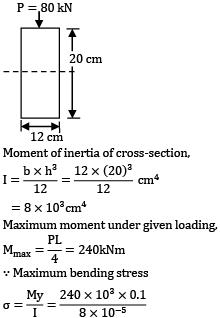Moment of inertia of cross-section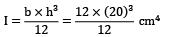= 8 x 103cm4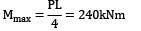Maximum bending stress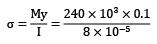= 300 MPa

QUESTION: 2

A beam with a rectangular section 120mm × 60mm, designed to be placed vertically is placed horizontally by mistake. The maximum stress is to be limited, the reduction in load carrying capacity would be

Solution: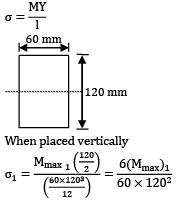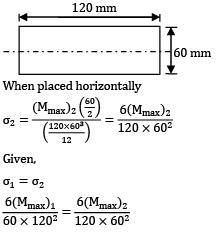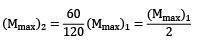So load carrying capacity would be half if placed horizontally by mistake in place of vertically.

QUESTION: 3

The ratio of the section moduli of a square beam (Z) when square section is placed(i) With two sides horizontal (Z1 ) and(ii) With a diagonal horizontal (Z2 ) as shown is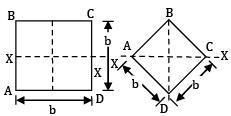Solution: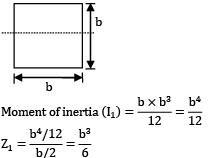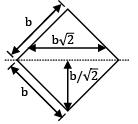Moment of inertia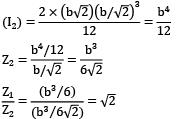QUESTION: 4

Two beams, one having a square cross-section and another circular cross-section, are subjected to the same amount of bending moment. If the cross-sectional area as well as the material of both the beams are the same, then

1. Both the beams will experience the same amount of deformation

2. The circular beam experience more extreme flexural stress than the square one

Which of the above is/are correct?

Solution: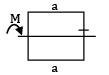Area = a2 = A, material is same this means that E is the same.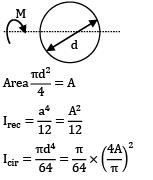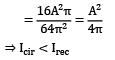Assuming same span length and loading beam having larger moment of inertia will have smaller deflection.

⇒ δrec < />cir

⇒ Statement 1 is wrong (Flexural strength)max = Mymax / 1 For the same bending moment, a beam having larger ymax / 1 will experience large flexural stress.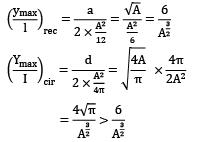⇒ Circular section experience more flexural stress.

QUESTION: 5

A cantilever of length 1.2m carries a concentrated load of 12kN at the free end. The beam is of rectangular cross-section with breadth equal to half the depth. The maximum stress due to bending is not to exceed 100 N/mm2. The minimum depth of the beam should be

Solution: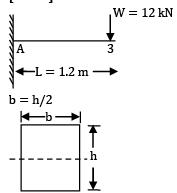At point A, Mmax = WL

= (12 × 103)N­m × (1.2)

= 14.4 × 103N­m = 14.4 × 106N­mm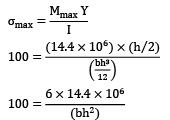bh2 = 6 × 14.4 × 104

(h / 2) × h2 = 86.4 × 104

h3 = 172.8 × 104

h = 120 mm

QUESTION: 6

A bar of rectangular cross-section (b × 2b) and another bar of circular cross-section (diameter = d) with the same length, are made of same material, and are subjected to the same bending moment and have the same maximum bending stress developed. The ratio of weights of rectangular bar and circular bar will be

Solution: Maximum bending stress in rectangular bar = Maximum bending stress in circular bar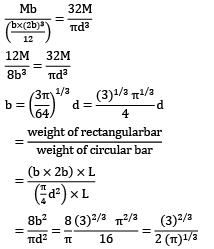QUESTION: 7

An equal flange I-section of 300mm depth and 200mm wide flanges has a uniform thickness of 10mm. it is subjected to a bending moment of 100kN-m. The bending stress at top most fiber is (in MPa)

Solution: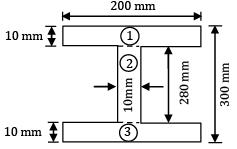Due to symmetry ̅X = 100 mm ̅

Y = 150 mm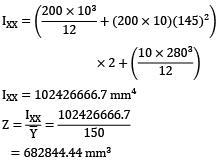Bending stress

M = fZ

100 × 106 = f × 682844.44

f = 146.44 N/mm2

QUESTION: 8

A cantilever has length of 2.5cm. It is of T section with IX = 2127cm4 and fibre distance (tensile) from neutral axis is 7cm. if the maximum allowable tensile stress is 300kgf/sq cm. Then the maximum UDL that can be applied in kg/cm is

Solution:

Bending equation,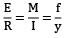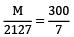M = 91.157.143kgcm

= 911.571kgm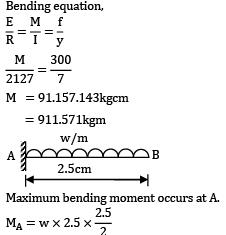MA = 3.125w

Substituting M value, 3.125 w = 911.571

w = 291.7kg/m

QUESTION: 9

A beam AB simply supported at its ends A and B, 3m long, carries a uniformly distributed load of 1kN/m over its entire length and a concentrated load of 3kN, at 1m from A.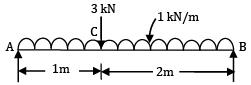If ISMB 150 with IXX = 300 cm4 is used for the beam, the maximum value of bending stress is

Solution: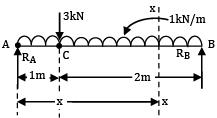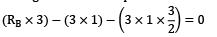3RB = 3 + 4.5

RB = 2.5 kN RA + RB = 3 + (1 × 3)

RA + RB = 6

RA = 3.5 kN

Mmax = (B M)c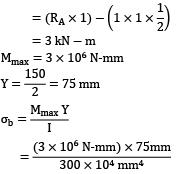= 75 MPa

QUESTION: 10

The maximum shearing stress induced in the beam section at any layer at any position along the beam length (shown in figure) is equal to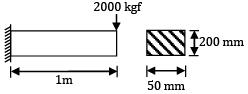Cross-section of beam

Solution: The shear cross section,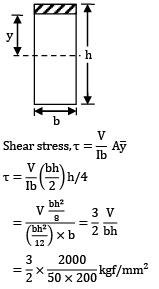= 30 kgf/cm2

QUESTION: 11

Simply supported beam of 3m span is subjected to loads as shown in figure below the beam is of I-section and all of the dimensions are in mm. The maximum compressive stress at point D in the web is (The section is located at a distance of 1m for right hand support)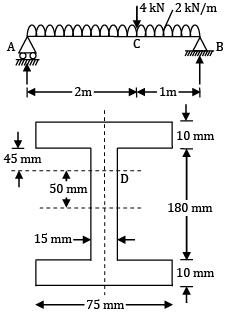Solution: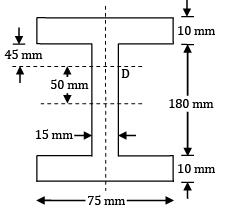Reactions, 2 × 3 × 1.5 + 4 × 2 = 3RB

17 = 3RB

Reactions, RB = 5.667 kN

Reactions, RA = 2 × 3 + 4 − 5.667

= 10 kN − 5.667 = 4.333 kN

MC = Bending moment at C

= 4.333 × 2 − 2 × 2 × 1

= 8.666 − 4 = 4.666 kN­m

= 4.666 × 106 Nmm

(Sagging) Shear force at C

FC = 4.333 − 2 × 2 = 0 = 0.33 3 (just left to C)

FC ′ = 0.333 − 4 = −3.667 kN (just right to C)

Jump shear force at C = 4 kN ↓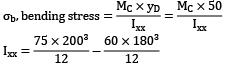= 50 × 106 − 29.16 × 106

= 20.84 × 106 mm4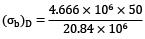= −11.20 N/mm2 (Sagging moment) compressive

Shear stress at D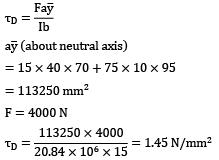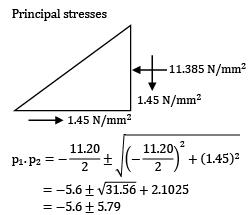p1 = 11.385 N/mm2

p2 = +0.19 N/mm2

QUESTION: 12

Two beams of equal cross-section area are subjected to equal bending moment. If one beam has square cross-section and the other has circular section, then

Solution: If, for the same applied moment, stresses generated are less, then the beam can be said as stronger.

Given, Asquare = Acircular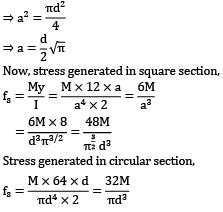Stress generated in circular section,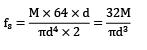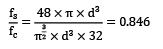⇒ Stress in square cross-section < stress="" in="" circular="" />

Hence, the square beam is stronger.

QUESTION: 13

A simply supported beam of span L and constant width b carries a point load W at mid-span. The depth of the beam required at the mid span for maximum extreme fiber stress P.

Solution: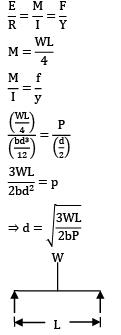QUESTION: 14

A link is under a pull which lies on one of the faces as shown in the figure below. The magnitude of maximum compressive stress in the link would be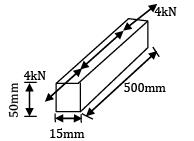Solution: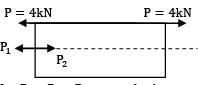Let P1 = P2 = P at neutral axis So P and P2 gives bending moment and P1 give axial tensile force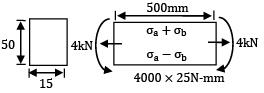Bending stress,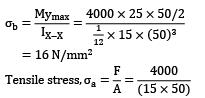Maximum compressive stress at lower fiber

= σb − σa

= (16 − 5.33)

= 10.67 N⁄mm2 = 10.7 MPa

QUESTION: 15

Beam A is simply supported at its end and carries UDL of intensity ‘w’ over its entire length. It is made of steel having Young’s Modulus E. Beam B is cantilever and carries a UDL of intensity w/4 over its length. It is made of brass having young’s modulus E/2. The two beams are of the same length and have the same cross-sectional area. If σA and σB denote the maximum bending stresses developed in beams A and B, respectively, then which one of the following is correct?

Solution: Bending stress in a beam is given by

σ = My / I

σ = M / Z

Where Z is section modulus For beams with the same cross section, Z is the same. It is given that the cross sectional area is the same. We cannot infer that Z is same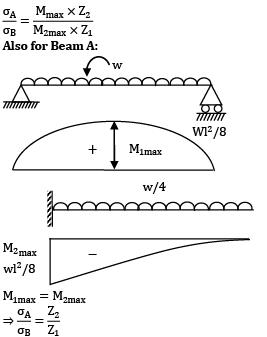Since, Z1 and Z2 depends on shape of cross section, hence σA / σB also depends on shape of

cross section.

QUESTION: 16

At a section of a beam, shear force is F with zero BM. The cross-section is square with side ‘a’. Point ! lies on the neutral axis and point B is mid-way between the neutral axis and top edge, i.e. at distance a/4 above the neutral axis. If τA and τB denote shear stresses at points A and B, then what is the value of τA⁄τB?

Solution: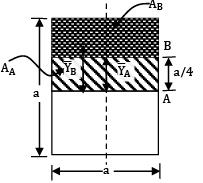For square beam, shear stress,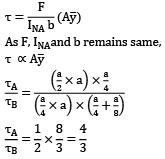QUESTION: 17

If the area of cross-section of a circular section beam is made four times, keeping the loads, length, support conditions and material of the beam unchanged, then the quantities (List-I) will change through different factors (List-II). Match the List-I with the List-II and select the correct answer using the code given below the lists.

List-I List-II

a. Maximum BM 1. 8

b. Deflection 2. 1

c. Bending Stress 3. 1/8

d. Section Modulus 4. 1/16

Codes: a b c d

Solution: A2 = 4 A1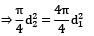Assuming, d2 = 2d1 A case of cantilever beam loaded with a point load P is used.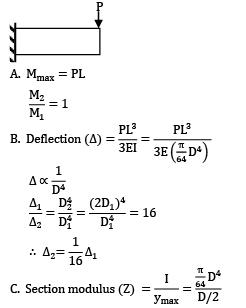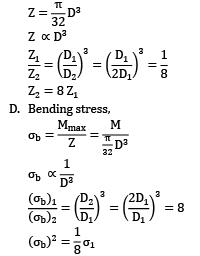QUESTION: 18

A shaft is subjected to torsion as shown in the figure below.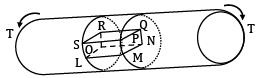Which of the following figures represents the shear stress on the element LMNOPQRS?

Shear stress

Solution: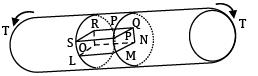When a shaft is subjected to torsion, shear stresses act over the cross-section and on longitudinal planes. This shaft is under pure shear whose direction depends upon the directions of the applied torque T.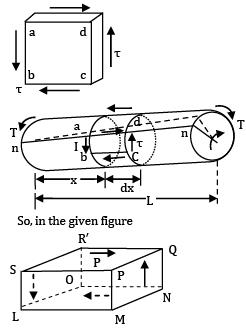So, in the given figure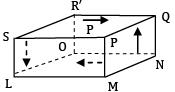QUESTION: 19

A cast iron water pipe 300 mm outer diameter with 15 mm wall thickness is supported over a span of 8 m. The maximum stress in cast iron when the pipe is running full of water is ___________MPa.

Take Density of CI = 71.6 kN/m3, Density of water = 9.8 kN/m3

(A) 12.9

(B) 13.7

Solution: Let us consider one meter length of water pipe CI pipe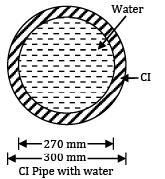Outer diameter, D = 0.3 m

t = 0.015 m

Inside diameter, d = 0.27 m

Area of cross-section (CI)

= π /4(0.32 − 0.272) = 0.01343 m2

Area of cross-section of water

=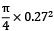= 0.05725 m2

Weight of CI per meter length

= 0.01343 x 71.6 = 0.9616 kN

Weight of water per meter length

=0.05725 x 9.8 = 0.5610 kN

Total weight of beam per meter length,

w = 0.9616 + 0.5610 = 1.5226 kN/m

L = 8 m

Maximum bending moment

Mmax =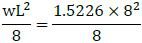= 12.1808 kNm

= 12.1808 × 106 Nmm

Section modulus of CI pipe,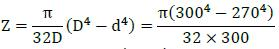= 91.158 × 104 mm4

Maximum stress in CI pipe,

σ =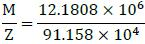= ± 13.36 N/mm2

Question_Type: 5

QUESTION: 20

The shear force diagram for rectangular cross section beam is shown in the figure below. Width of the beam in 100 mm and depth is 250 mm, the maximum bending stress in the beam is ___________ MPa.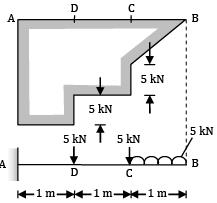(A) 25.8

(B) 27.0

Solution: SF diagram is only on one side. This is the case of a cantilever 3 m long AB, fixed at A and free at end B carrying UDL of 56 kN/m over BC = 1 m, a point load of 5 kN at C and a point load of 5 kN at D. Leading diagram is shown in the figure below.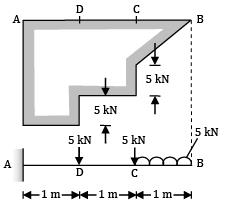Maximum bending moment occurs at fixed end A

MA = 5(2.5) + 5 × 2 + 5 × 1

= 12.5 + 10 + 5 = 27.5 kNm

= 27.5 × 106 Nmm = σb × Z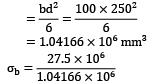= 26.4 N/mm2 (Max. bending stress in beam)Use Code STAYHOME200 and get INR 200 additional OFF Use Coupon Code

Track your progress, build streaks, highlight & save important lessons and more!

Similar ContentRelated tests